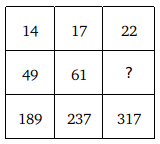Question 6

# Study the given pattern carefully and select the number that can replace the question mark(?) in it.Solution

14 $$\times$$ 4 - 7 = 49, 49 $$\times$$ 4 = 196 - 7 = 189
17 $$\times$$ 4 - 7 = 61, 61 $$\times$$ 4 = 244 - 7 = 237
Similarly,
22 $$\times$$ 4 - 7 = 81, 81 $$\times$$ 4 = 324 - 7 = 317
$$\therefore$$ The correct answer is option D.

• Free SSC Study Material - 18000 Questions
• 230+ SSC previous papers with solutions PDF